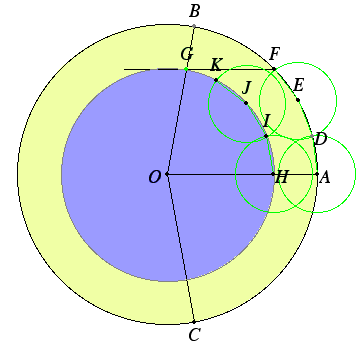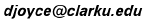### Duplicate the cube?

An idea for duplicating a cube. The problem is this. Given a cube of volume a2, construct a cube of volume 2a2. More precisely, construct a side b of such a cube. In more modern terminology, give a length a, constuct a length b so that b/a is the cube root of 2. If you set the length of a to be one, then the problem is just to construct a line whose length is the cube root of 2. The limitation is that this must be done with Euclid's tools of plane geometry, the straightedge and compass.

Here's one proposed solution. Construct an isosceles triangle with base BC equal to 1 and height AD equal to 2.

Trisect the base BC at points E and F, that is, make BE = EF = FC, each of length 1/3. Then connect E and F to the vertex A.

Let G be the midpoint of the side AB. Construct an isosceles triangle BGH with H a point on the other side AC, and BG = BH.

Let GH intersect AE at L, and GH intersect AF at J. Also, let BH intersect AE at O, and BH intersect AF at N.

Then draw lines parallel to GH through N and O, and let them intersect AB at K and I, respectively. Also draw lines KL and IJ parallel to BH.

The three lines IJ, AE, and KN meet at a point. (They look like they do,anyway.)It was proved in the 19th century that a cube can not be duplicated with the Euclidean tools of straightedge and compass.